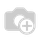# How to compute Purchase Order Line field and show in Purchase Order field

i create a custom field x_line_total in Purchase order Line and create another custom field x_grand_total for show sum of x_line_total  field. How to compute x_line_total field into x_grand_totalfield?

This Code i written on .py file but this is not correct

class PurchaseOrder(models.Model):
_inherit = "purchase.order"

@api.depends('order_line.x_line_total')
def _compute_x_grand_total(self):

for line in order.order_line:
x_grand_total += line.x_line_total

x_grand_total = fields.Float(compute='_compute_x_grand_total', string='Grand Total')Hi!

Change the code like this :

`class PurchaseOrder(models.Model):    _inherit = "purchase.order"        x_grand_total = fields.Float(compute='_compute_x_grand_total', string='Grand Total')    @api.depends('order_line')    def _compute_x_grand_total(self):        self.x_grand_total = sum(line.x_line_total for line in self.order_line)`

Best regards!